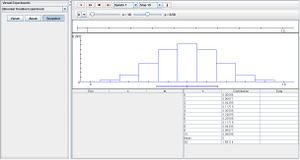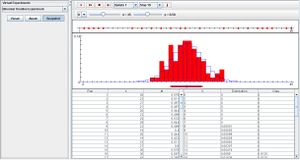# SOCR EduMaterials Activities BinomialTimelineExperiment

## Description

Demonstrating the characteristics of Bernoulli trials, the binomial timeline experiment performs n trials with the fixed probability of success p. For every trial, the successes are recorded as red dots on a timeline with interval 1 to n. Under the timeline displays one graph that illustrates the discrete probability density function and moments of the selected variable as blue and the empirical probability density function and moments of the selected variable are shown in red. Located under the graph at the bottom left of the page is the data table that records every run with the number of successes X and the proportion of successes M. To the right of the data table is the numerical values of the distribution table that also updates the mean and standard deviation of the gathered data in the experiment. Either X or M may be selected with the list box; note that X is the initial selection. The parameters n and p can be modified with the scroll bars above the timeline.

## Goals

To provide a method illustrating the unbiased phenomenal events that occur during a given period of time (e.g. rainfall in a week, peak temperature during the day, etc.) and give a generalization about these natural events.

## Experiment

Go to the SOCR Experiments [] and select the Binomial Timeline Experiment from the drop-down list of experiments on the top left. The image below shows the initial view of this experiment:When pressing the play button, one trial will be executed and recorded in the distribution table below. The fast forward button symbolizes the nth number of trials to be executed each time. The stop button ceases any activity and is helpful when the experimenter chooses “continuous,” indicating an infinite number of events. The fourth button will reset the entire experiment, deleting all previous information and data collected. The “update” scroll indicates nth number of trials (1, 10, 100, or 1000) performed when selecting the fast forward button and the “stop” scroll indicates the maximum number of trials in the experiment.

Note that when increasing the value of parameter n, the discrete probability density function graph takes shape of a normal curve. Increasing parameter p shifts the graph right and decreasing it shifts the graph left. When p is set to its extremities, the graph will no longer take shape of a normal curve. As the number of runs increase, the empirical graph begins to take shape of the discrete graph.## Applications

The binomial timeline java applet may assist those who are interested in determining events that may occur in a given period of time.

For example, if the event of interest is a given day with great beach weather during spring break, one may use this applet once the probability of great beach weather for one day (success) has been obtained.

Suppose astronomers want to use this applet to determine the probability of a meteor shower (success) in the next one hundred years.

A group of students are interested in the probability of their teacher giving them a pop quiz one month before finals week.